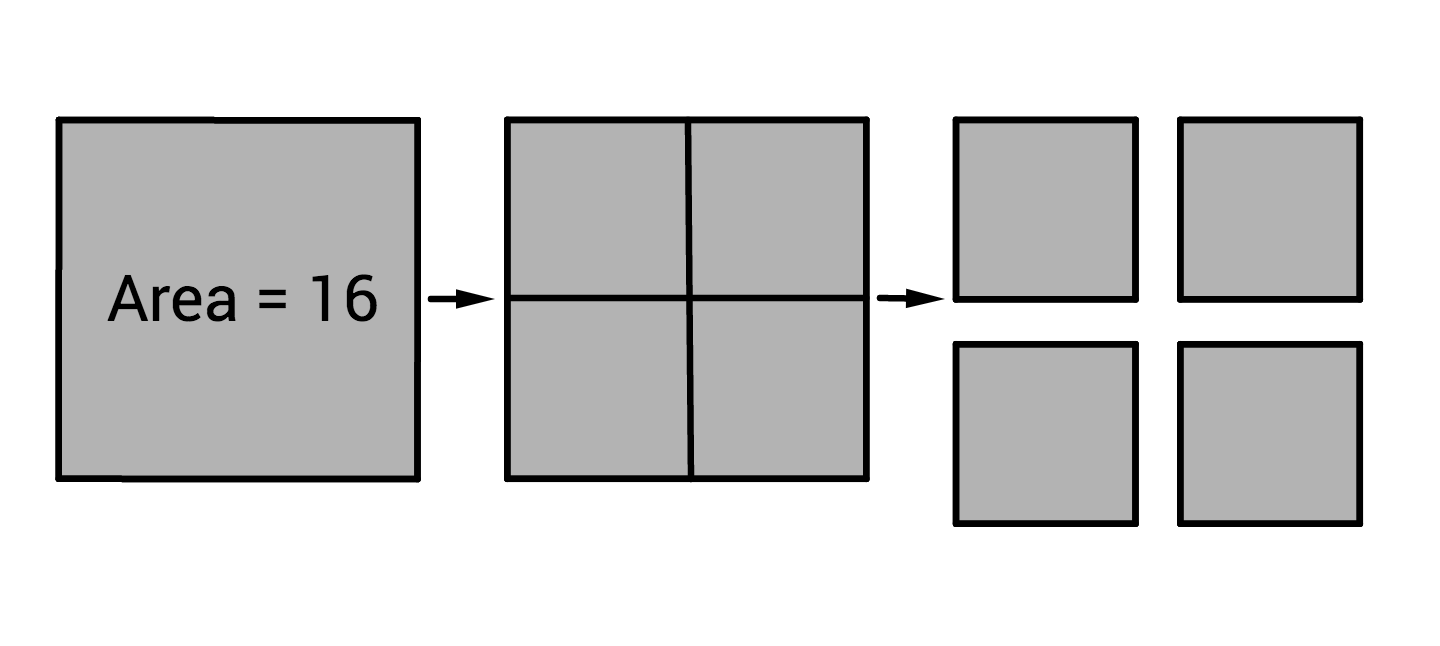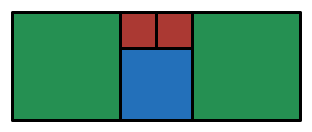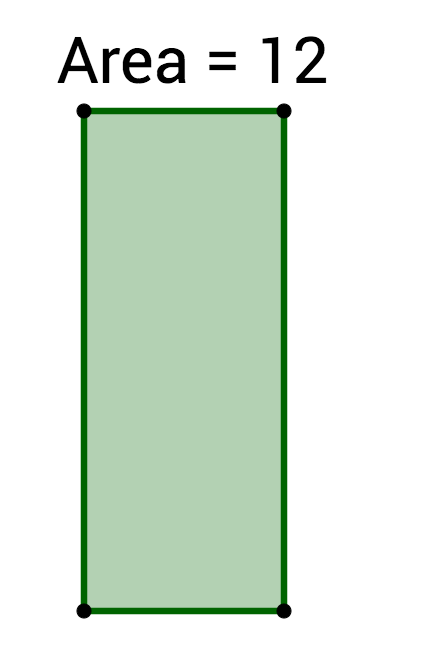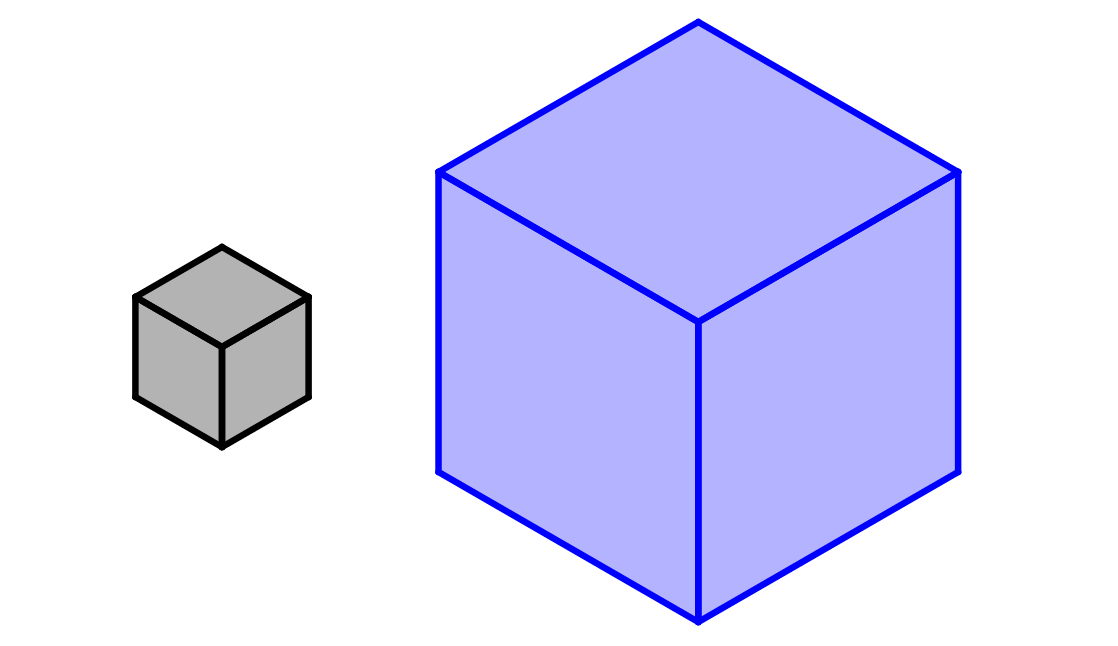Everyday Math

# Length, Area, and VolumeThe area of a square is 16. It is cut into four smaller congruent squares. What's the combined perimeter of the four smaller squares?The above image shows a collection of squares. If each red square has a side length of 1 unit, what is the area of the entire figure?The rectangle has an area of 12. If we halve the length and the width, what will be its new area?The small grey box has a volume of 2 and is enlarged equally in all dimensions to create the large blue box, whose volume is 54. How many times longer are the side lengths of the blue box?

A square and a regular polygon both have a perimeter of 24. If the side lengths of the square are 3 units longer than the side lengths on the polygon, how many sides does the polygon have?

×